Select PageLet us return to viewing this essential odd-even split in the alef-bet as a proportion. We can draw them as two adjoining segments on a line, two adjacent rectangles, or even as we have represented them in the 3-d bar graph below. The important thing is the proportion, not the shape that is being split apart; it is the relationship between the two shapes.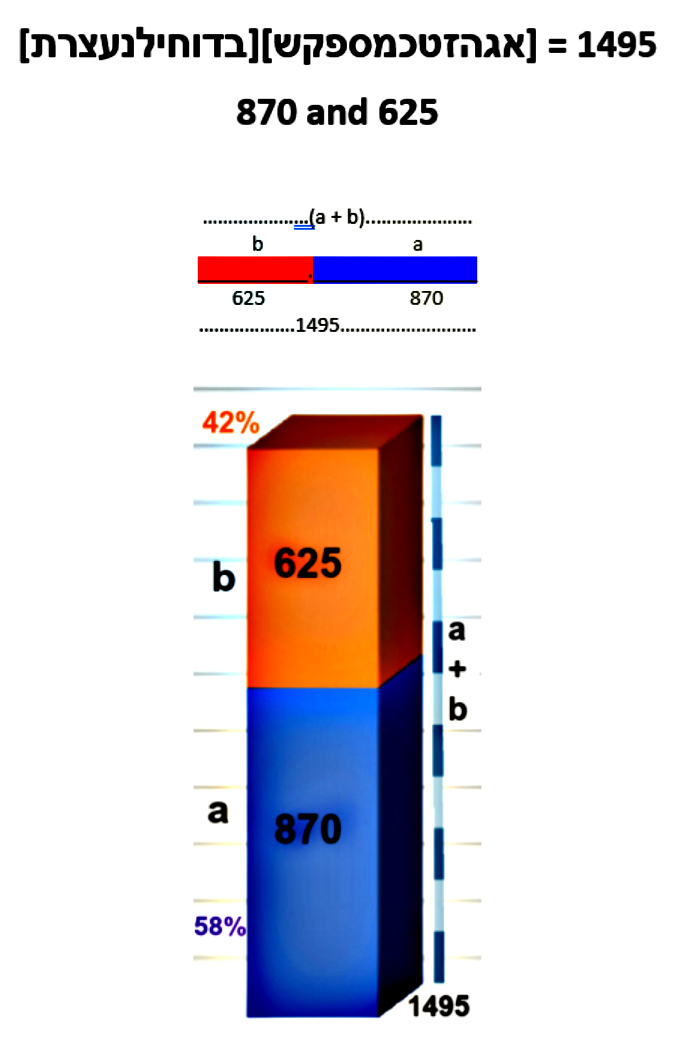We know that the odd set is 72% of the even set and that the odd set is 42% of the whole set of letters. Mathematically, we write this as b : a = .7180 and b : c = .4180

b : a = .7180

b : (a+b) = .4180

What is this reminiscent of? The Golden Mean, Phi().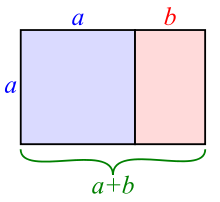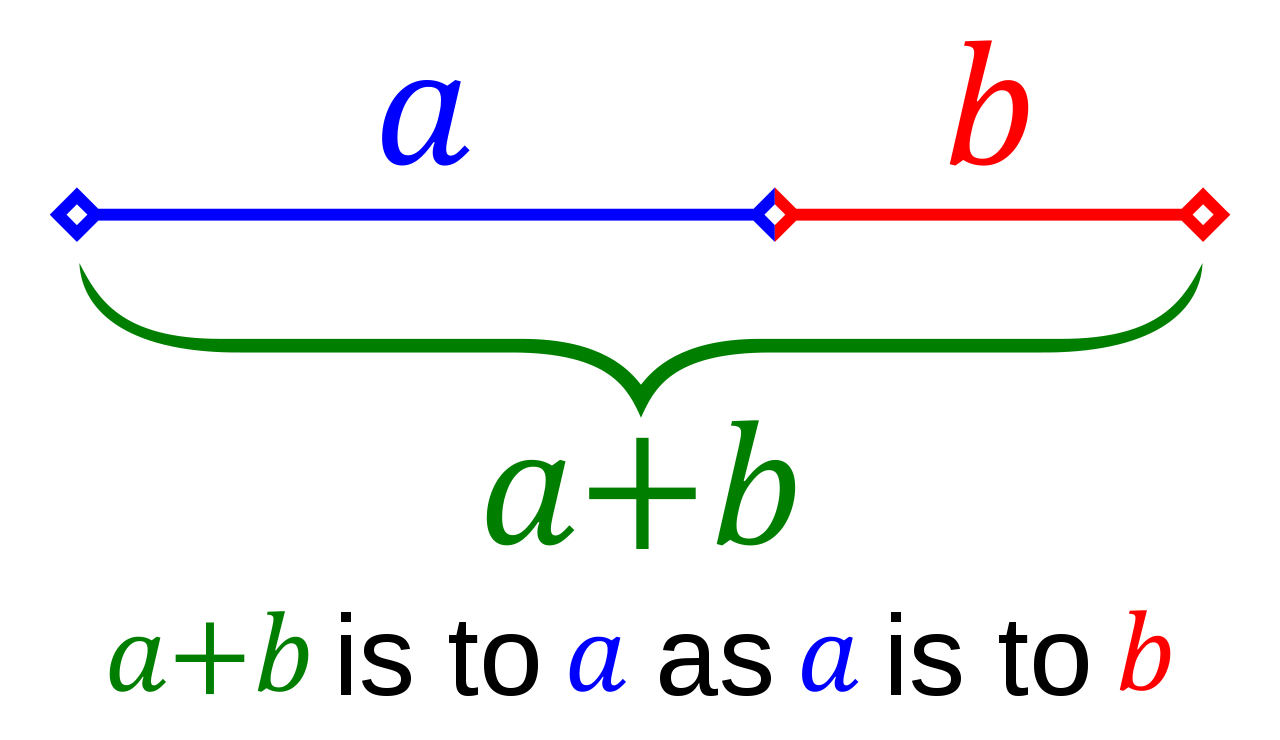b : a = .6180
a : (a + b) = .6180
The relationship between the segments a to b and between the segments b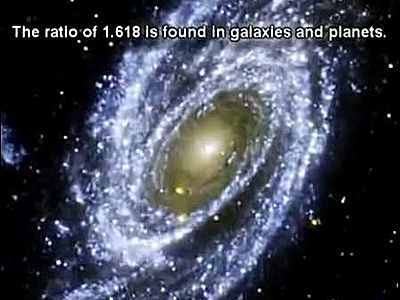to (a + b) is highly reminiscent to the harmonic golden mean, the Phi () proportion. Phi() is a mathematical constant, an irrational number that naturally and automatically guides the most efficient and beautiful spiraling growth in the universe.  This includes flower petals, seashells, tree branches, the shape of our bodies, our DNA, the galaxies in the universe and several different aspects of black holes.  It is not a stretch to say that without Phi() chaos would truly reign in our universe, as will be explained later. For now, just picture your DNA instead of following neat exact double helix spirals, as random knots, or the petals on a flower as a jumbled mess.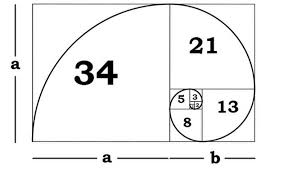Quite simply, the Phi () proportion is such that the small segment (b) is to the larger one (a) as the larger one is to the overall length (a + b). This proportion is called Phi () and is equivalent to .61803399….. The spiraling growth happens by continually dividing the larger segment by .618033…, or alternatively multiplying it by 1.618033…
If we were to apply the Phi () proportion to the 1495 total value of the alef-bet, the subordinate values would be split into 924 + 571 = 1495, where a = 924 and b = 571.

1495 x .6180 = 924

924  x  .6180 = 571

1495

Comparing the Phi () proportioned alphabet to the odd-even split we can see that it is an exact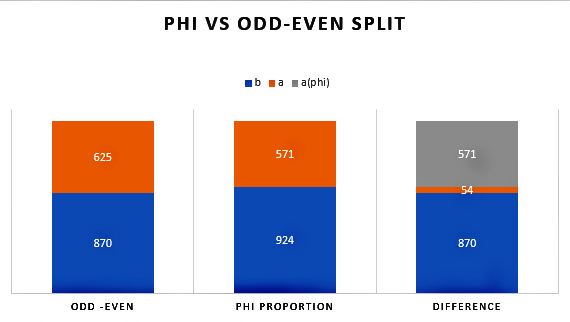shift of 54 units from Phi () to the Odd/Even split.

625 – 54 = 571

870 + 54 = 924

Why so exact? If we review the proportions, we recall that:

625/1495 was .4180

625/870 was   .7183

They are off from Phi (), .6180 by a pretty round number:

.6180 – .4180 = .2000

.7183 – .6180 = .1003

### A Connection to Power and the Torah’s First Verse

Oddly enough, when we add the digits in the odd/even split we get:

6 + 2 + 5 + 8 + 7 + 0 = 28

We also get 28 when we add the digits in the Phi() split:

5 + 7 + 1 + 9 + 2 + 4 = 28

And again, when we add the total value of the alef-bet plus the shift of 54 units:

1 + 4 + 9 + 5 + 5 + 4 = 28Summing up the digits may seem like child’s play compared to the rest of the mathematics we are discussing, yet it is a typical part of Number Theory, which is one of the main branches of mathematics.  As will be obvious soon, the number 28 here relates to the 28 letters in the Torah’s first verse. The fact that all three equations, dealing with the four different components, sum to 28 is not slight of hand. There is no reason mathematically for them to work out that way; it must either be a tremendous coincidence or purposeful design.  The beauty of Number Theory is that it deals in hard facts and can reveal and/or disprove wonderful symmetries in the framework of our universe.
The same question—a tremendous coincidence or purposeful design—can similarly be applied to the sum total of the standard gematria value for all 304,805 letters in the Torah: 21009826. The sum of its digits (2+1+0+0+9+8+2+6) = 28, the same result (28) that we saw in the equations above.  Also, the product of those same digits gives us (2*1*0*0*9*8*2*6) = 1728.  Then applying another deeply revealing form of gematria cipher by dividing the above product by the above sum, we get:

##### 4√(P(21009826)/ ∑(21009826)) = 4√(1728/28) = 2.802828

This is not so dissimilar from the square root of the exact number of words in the Torah: 282.8002828
You see, pure facts, just like 2 + 2 = 4, revealing beautiful symmetry. Though this does not prove the existence of G-d, it does go a long way in proving purposeful design, which can be taken as divine intent. Now some scholars in both Number Theory and ancient Kabbalistic gematria studies would go one step further in all these equations and reduce 28 to 2 + 8 = 10, which further reduces to 1 + 0 = 1.  More on that later.  In case you were wondering, the number 28 is the gematria value for the Hebrew word Koach(כח), meaning “power.”
Here are a couple more pure facts about the total gematria of the Torah. If you recall, we found the square root the total gematria sofit value (33640626) of the 304,805 letters in the Torah is 5800.054, which was astonishing considered that exact collective number of words, letters and verses in the Torah is exactly 58. Nevertheless, the gematria sofit value and the standard gematria (21009826) value are also tied to those of 58 quantitative (or structural) elements of the Torah through its square root, 625, which also represents the proportion 5/8 = .625:

21009826/33640626 ≈ .625 = 5/8.

Moreover, while the sum of the 22 spelled out Hebrew letters is 4248, it becomes 10048 when the final letters are considered in the spelling, in other words exactly (10048-4248) = 5800 more.  Oh, and by the way, (3+3+6+4+0+6+2+6) + (2+1+0+0+9+8+2+6) = 58.  Did any of this have to work out this way? No. Could it have happened accidentally, by random chance? No. Well yes, there is exactly one way it could work out by random chance and that is if there exists an infinite set of varying universes and we just happen to live in the One that everything concerning our Torah, and as you will soon see, everything concerning our mathematical and physical constants, our mathematical constructs and the dimensions of our solar system also happen to work out with perfect symmetric harmony.  Either way, it makes us pretty special.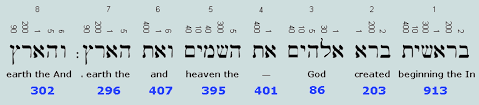Nonetheless, back to that first 28 letter verse of the Torah, the first 5 letters (בראשי) of that verse and thus of the Torah have a collective ordinal value, letter placement within the alphabet value, of (2+20+1+21+10) = 54.
Please bear with me as I reveal yet another secret code embedded into the Torah. I know it is out of place, but I just noticed a heretofore unforeseen pattern and cannot contain my excitement. If we plot out the ordinal values of the first 11 letters of the Torah. Notice that it is 11 letters again, and please note that there are exactly 11 unique numbers in that same first verse. A hint in unraveling the Torah encoding is that whenever there is abundant redundancy, someone is trying to communicate a message to us and that it is important enough to ensure we do not miss it. Plotting out those 11 letters, we get:

# בראשית־ברא־אל

### 2,20,1,21,10,22,2,20,1,1,12 and their sum is 112.

First, we combine the letters so you can see what I was seeing:

### 22012110222201112

Then we break them down to natural viable triplets:

### BRE  Sh  Y T      BRE   EL

If because all these letters just happened to work out to 0’s, 1’s and 2’s we can then convert this message from base 3 to base 10, giving us:

### 73  7 3 8 73 14

Permuting the letters slightly we get:

### BRE  YSh  T   BRE   EL

Which translates to “G-d created Something 22”, implying not only G-d Created the 22 letters, but moreover, the deep kabbalistic concept of “Something from Nothing” that we will get into later.

### BRE  Y – Sh – T   BRE   EL

We already know that the entire first verse totals 2701, which is the sum of all the integers from 1 – 73 and that 73 is the gematria of Cochma(חכמה), meaning “Wisdom,” and the second highest of the 11 dimensions. That total 2701 also equals 73 x 37.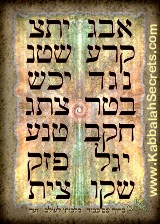If we broke the word sequence into its natural triplet sequencing (BRE, ShYT, BRE, EL) we would get (7+3+6+0+2+7+3) = 28, as is also (BRE YSH T = 7+3+7+3+8).  Meanwhile, there are 9 digits in (737387314) and 9 divided by the small gematria of the 42-Letter Name is 9/.123049… = 73.141593… combining 73 and Pi to an astonishing degree of accuracy. This 314 is also found in the Torah’s first word, (שית), again as the small gematria value (314). While it is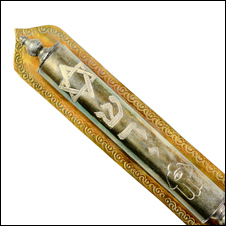obviously indicative of Pi (3.14…) it is also representative of the Name of G-d, Shadai (שדי) that we put on our doorposts, gateways and entrances.  That is all we are going to say about this today, the gateway to Wisdom and Knowledge. Moreover, reinforcing the “G-d Created Something 22”:

##### 2+2+0+1+2+1+1+0+2+2+2+2+0+1+1+1+2 = 22 (letters)

Also, YeSh (יש) in base 6 is 54 and base 8 is 42, while in base 13 it is 28, all very relevant as we will soon see, as is Y- SH-T (י־ש־ת) or 378, the value of the famous phrase “Echad Ushmo Echad (אחד־ושמו־אחד)”  or “His Name is One.”
Yet if we kept things simple, and just used the 11 ordinal values as their individual base 10 equivalents, we would get:

## 2 + 6 + 1 + 7 + 3 + 8 + 2 + 6 + 1 + 1 + 5 = 42

We would have also seen 26, representing the Tetragrammaton (יהוה);
173, representing the small gematria value of the 42-Letter Name and the 173 Keys to Heaven the 1900-year-old Zohar tells us were given to Moses; and 26 yet again. And in all fairness to gematria buffs, 115 is the value of hazak (חזק), the Hebrew word for strength.
We digressed again, and yet it is interesting how this phenomenon of 28 connects to the resultant ratio: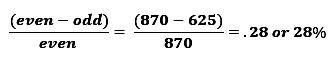### Phi and 54-Unit Shift

The shift between the odd-even split of the alphabet and the Phi() one is exactly 54 units, so reminiscent of the 54 portions in the Torah.  Meanwhile, the 54-unit shift represents 5.844% of the larger Phi() split (924), which is so close to the 5845 verses in those 54 portions.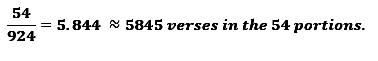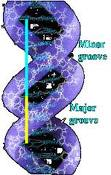That the Torah’s 54 portions and 5845 verses would be connected to Phi() is not surprising, considering that the first chapter of the Book of Numbers (Bamidbar) is the dividing point in the Torah in terms of the number of letters, the numbers of words, and also the number of verses into the exact Phi() proportion, just like the major and minor twists of our DNA: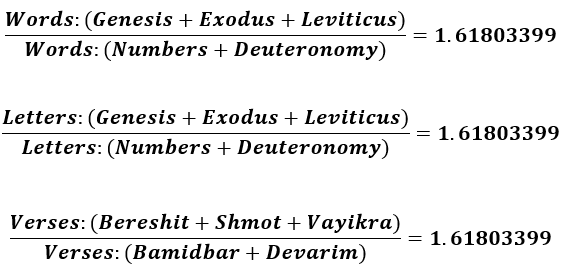Moreover, oddly enough, that first chapter of Bamidbar, where that telling split in the Torah occurs, has 54 verses, a wonderful synergistic connection to the 54-unit Phi() shift. The first verse of the Torah is also split into two sections that incorporate the number 54. We already illustrated a connection between 73 x 37, now we see that the last two words of that verse have a joint gematria value of 703, which is 19 x 37, while the first 5 words have a gematria of 1998 or 54 x 37.
What all these numbers have in common is that they all reduce to One (1).

73 →7 + 3 = 10  →   1 + 0 = 1 (One)

37→3 + 7 = 10  →   1 + 0 = 1 (One)

2701→ 2 + 7 + 0 + 1 = 10 →   1 + 0 = 1 (One)

19 → 1 + 9 = 10  →   1 + 0 = 1 (One)

(54 x 37) → 5 + 4 + 3 + 7 = 19 → 1 + 9 = 10 → 1 + 0 = 1 (One)

(19 x 54) → 1 + 9 + 5 + 4 = 19 → 1 + 9 = 10 → 1 + 0 = 1 (One)

And

541 (Israel) 5 + 4+ 1 = 10  →  1 + 0 = 1 (One)

424 (Moshiach Ben David) 4 + 2 + 4 = 10  →  1 + 0 = 1 (One)

The number 37 is in actuality 111/3 or the numerical value of Alef/3 so theGenesis 1:1 equation above translates to: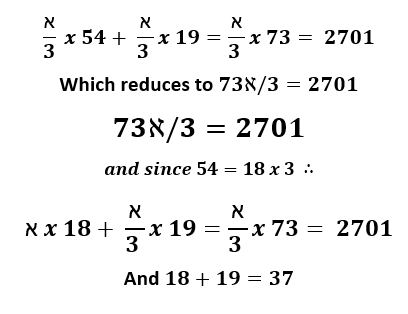Much has been written about the unique geometry of this first verse, though the only one we will mention today is that 37 and 73 are both consecutive 12-gonal numbers, meaning they form a filled in Magen David, Star of David.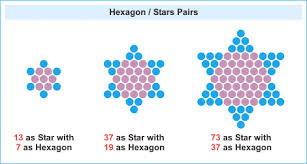The first two Star numbers are 1 and 13–harking back to Echad (One)–of the star number sequence is 1, 13, 37, 73…253…541, 661…. Most fittingly, the number 541, the numerical value of Israel, is the 10th Star number, while the 11th Star number, 661, is the gematria of Ester, the heroine of Purim, in which by the way, there are exactly 54 Hamans, her adversary in the Scroll of Ester.  Interestingly, the 7th Star number, 253, is the sum of all the integers from 1 – 22, as in the 22 letters.  Moreover, we have already explained that 73 is the value of the sefira Chochma, meaning Wisdom, not we can reveal that 37 is the ordinal value of Chochma, or inner Wisdom, which geometrically makes sense since because the central hexagon within the Star of 73 is 37.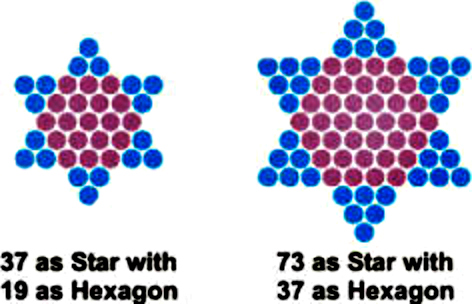Another connection between 19 and 54 and 73, besides 19/73 = .260, the numerical value of the Tetragrammaton (יהוה), is that 19 of the 54 portions of the Torah begin with a word that has either a gematria value, or an ordinal value, of 42. One final redundant connection is that 54 is the sum of the small gematria of the first 5 words of the Torah, while the next two words of the 7-word first verse is 28. The first word is 13.
Before we return to the relationship between the alef-bet and Phi proportions, there are two more equations at the heart of the Torah that establish the indelible connection between Phi and the Torah and illustrate the degree of precision and intent that went into designing the Torah. As first revealed in The Genesis Prayer: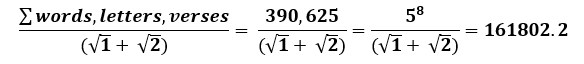If we add the 248 columns in the Torah and the actual number of total rows in the Torah (248 x 42 rows/column less all the blank rows) to the total words, letters and verses, we get a total of 401273 for the 5 separate written divine elements in the Torah, and: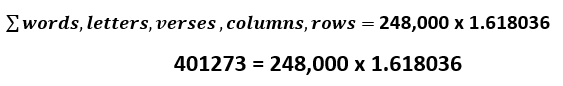How close is that to true Phi? It is about 99.9999% precise, or within 1.0000012 of actual phi.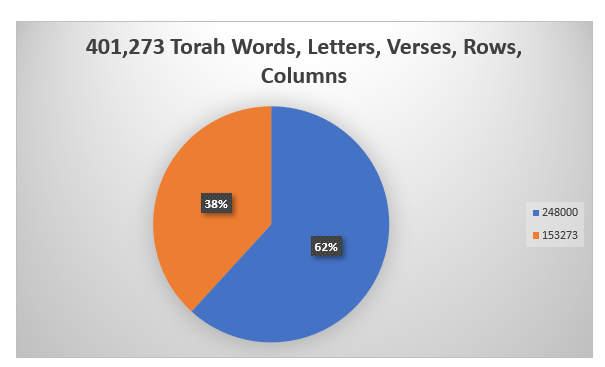Why 248 and 248,000? We do not know, except that 248 is the gematria of Abraham and the sum of the Hebrew Names of each of the 5 Books is 2480, and that there are 248 columns in “The Torah(התורה)” of numerical value 616, whose square root is 24.8….  Maybe it is another way of pointing us in the direction of the 3 portals (1st Shema verse, 1st Torah Verse and 42-Letter Name), whereby 10,000 – their sum (1118 + 2701 + 3701) = 2480 yet again. Moreover, it may be connected to the fact that 2480 = 620 x 4 and that 620 is both the numerical value of Keter(כתר), the highest crowning sefira (dimension), and is representative of the 620 letters in the 10 Commandments, the high point of the Torah. Or maybe it is a deeper way to illustrate to us the connection between the Phi based E 8 Lattice of 248 dimensions and the 58 Torah elements.
Since we have shown that all Torah knowledge tends toward and point to Alef (One) it is not surprising that 248/5 = 111 (Alef), especially considering that the square root of 5 is the key component in Phi. And along those same lines, 8/5 = 3.58, as in the numerical value of Moshiach (358), the Anointed One.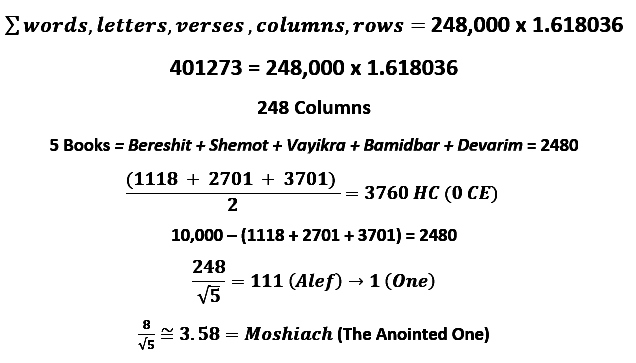### Hashem

The word for Name in Hebrew is Shem (שמ) and as we keep circling back to the importance of the connection between the 42=Letter Name, the Torah’s first verse and the Shema, it is noteworthy to mention, as Danny recently showed me, that the sum of the 4 Shins (ש) and 4 Mems (מ) in the Torah’s first verse and in the Shema’s first verse equals 2480, so the 8 Letters  = the 5 Names. Always symmetry, always redundancy, always a reason—it is our job to find it.
Coupled with Name in Hebrew, Shem (שמ), the letter Hey (ה) of numerical value 5, turns Shem into Hashem(השם), referring to G-d. The 5th Name of the 72 Names (Triplets) is (מהש), a permutation of Hashem(השם) and of Moses(משה), as in the 5 Books of Moses. The 5th word in the Torah, H’Shama’im (השמים), meaning Heaven, begins with Hashem(השם), so Heaven can be interpreted as the Sea of Hashem. Five (5) x 345, the numerical value of Hashem and Moses is 1775, the total gematria of the 27 letters of the Hebrew alphabet when the 5 final letters (ךםןףץ) are included. This is also the reason why Moses was accused of stealing the 1775 shekels collected for the building of the Mishkan in pasha Pekudei.
The association between 5 and the 5 Books of Moses and the 54-unit shift and the 54 portions of the Torah is deeper than just being integrated design elements meant to show off the Torah’s Creator’s knowledge of mathematics and the universe.  It has to do with how the Torah is split up, or partitioned, which we’ll get into shortly.  For now, just keep in mind that every Torah element must be divided 5 ways and also 54 ways.
Shavua Tov
The next installment continues shortly as we move out of the introductory phase of this series and into the heart of the matter.